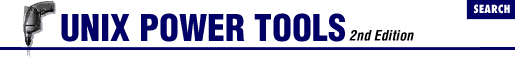home | O'Reilly's CD bookshelfs | FreeBSD | Linux | Cisco | Cisco Exam

#Chapter 49Working with Numbers## 49.5 Base Conversion Using cvtbase

cvtbase The bc command can be used to convert between different bases (49.2 ) , but an easier tool to use is cvtbase . cvtbase is designed specifically to convert from one format to another. For example, to convert a decimal IP address into its hexadecimal equivalent:

```% ```cvtbase d h
```

```140.186.65.25
```

8c.ba.41.19```

The first argument, `d` , means that our input will be in decimal form. The second argument, `h` , means that we want the input converted into hexadecimal. In general, the syntax for calling cvtbase is:

```cvtbase ```input_base output_base
```
```

where input_base and output_base are any of:

`d, D`

Decimal; i.e., digits `0` through `9` .

`x, h`

Hexadecimal (using lowercase); i.e., `0` through `9` and `a` through `f` .

`X, H`

Hexadecimal (using uppercase); i.e., `0` through `9` and `A` through `F` .

`o, O`

Octal; i.e., digits `0` through `7` .

`b, B`

Binary; i.e., digits `0` and `1` .

Any input characters that aren't in the specified set are sent through unchanged. In the example above, the dots (`.` ) in the IP address are retained in the hexadecimal output.

- LM49.4 bc's Sine and Cosine Are in Radians49.6 Quick Arithmetic with expr